## Introduction

Part 1 of this series explored the applications of absorbing Markov chains in the context of a simple perfect maze. This part of the series will expand on the simple maze in various ways and explore how these changes affect interpretations of the different metrics offered in the samc package.

The complete code for this series is available on Github.

## Setup

Part 2 reuses the code from Part 1.

## Fidelity

The first change explored in this part will be the incorporation of fidelity into the samc object. In Part 1, transitions always occurred from one cell to a different neighboring cell. With fidelity, transitions can occur from a cell to itself; essentially, there’s no movement during a time step. There are potentially many different ways that fidelity could be applied to the maze, but for simplicity, this example will keep things simple by using it to create a delay in movement at intersections. The goal is to model “hesitation” when an individual is presented with the choice of three or more paths. For additional simplicity, all intersections will be treated the same and assigned a fidelity probability of 0.1, which means that once an individual is at an intersection, there is a 10% probability that they will stay in the intersection from one time step to the next.

# Intersections determined using a moving window function
ints_res <- focal(maze_res,
w = matrix(c(NA, 1, NA, 1, 1, 1, NA, 1, NA), nrow = 3, ncol = 3),
fun = function(x) {sum(!is.na(x)) > 3})

ints_res[is.na(maze_res)] <- NA
ints_res <- ints_res * 0.1

plot_maze(ints_res, "Intersections", vir_col)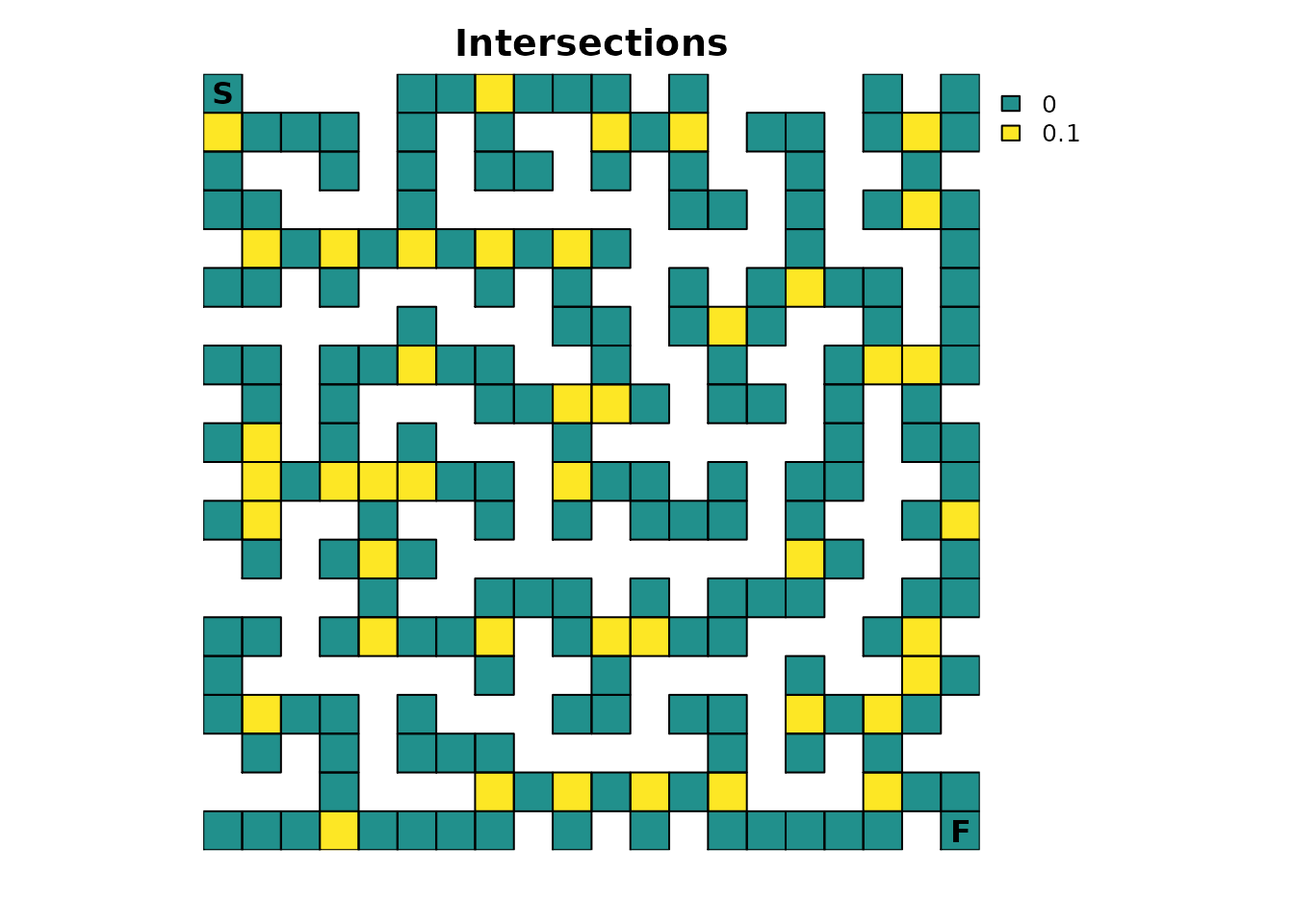Fidelity changes the $$P$$ matrix underlying the samc object, which means that the samc object has to be recreated:

ints_samc <- samc(maze_res, maze_finish, ints_res, model = rw_model)

To start, let’s see how the new fidelity input affects the expected time to finish:

# Original results from Part 1
survival(maze_samc)[maze_origin]
#>  13869
cond_passage(maze_samc, origin = maze_origin, dest = maze_dest)
#>  13868

# Results with fidelity at intersections
survival(ints_samc)[maze_origin]
#>  14356
cond_passage(ints_samc, origin = maze_origin, dest = maze_dest)
#>  14355

Intuitively, with “hesitation” added to the movement, the expected time to finish increases. Also, note that incorporating fidelity in this particular example does not affect the relationship between survival() and cond_passage().

In terms of the probability of visiting any particular cell, changing the fidelity does not change the results from Part 1:

ints_disp <- dispersal(ints_samc, origin = maze_origin)
#>
#> Performing setup. This can take several minutes... Complete.
#> Calculating matrix inverse diagonal...
#>
Computing: 100% (done)
#>
Complete
#> Diagonal has been cached. Continuing with metric calculation...

all.equal(maze_disp, ints_disp)
#>  TRUE

Fidelity does, however, change the number of times each cell is expected to be visited:

ints_visit <- visitation(ints_samc, origin = maze_origin)

all.equal(maze_visit, ints_visit)
#>  "Mean relative difference: 0.03511428"

# Let's plot the difference to see if there is a noticeable pattern
visit_diff <- map(maze_samc, ints_visit) - map(maze_samc, maze_visit)
plot_maze(visit_diff, "Visits Per Cell (Difference)", viridis(256))With fidelity present, the intersections are seeing a significantly different number of visits. Since a “visit” effectively represents a transition to a cell from one time step to the next, the presence of fidelity means that the metric is counting transitions from a cell to itself as well. Interestingly, when compared to the figure in Part 1, the legend in this figure seems to indicate that the increase for the intersections might be 10%, or the same as the fidelity probabilities. It also seems like the non-intersections (cells with a fidelity probability of 0.0) experienced no change. Let’s check these ideas:

# First, let's see which cells changed.
# Ideally would just use visit_diff > 0, but floating point precision issues force an approximation
plot_maze(visit_diff > tolerance, "Visits With Non-Zero Difference", vir_col)

# Second, let's see what the percent change is for our non-zero differences.
visit_perc <- (ints_visit - maze_visit) / maze_visit
visit_perc[visit_perc>tolerance]
#>   0.1111111 0.1111111 0.1111111 0.1111111 0.1111111 0.1111111 0.1111111
#>   0.1111111 0.1111111 0.1111111 0.1111111 0.1111111 0.1111111 0.1111111
#>  0.1111111 0.1111111 0.1111111 0.1111111 0.1111111 0.1111111 0.1111111
#>  0.1111111 0.1111111 0.1111111 0.1111111 0.1111111 0.1111111 0.1111111
#>  0.1111111 0.1111111 0.1111111 0.1111111 0.1111111 0.1111111 0.1111111
#>  0.1111111 0.1111111 0.1111111 0.1111111 0.1111111 0.1111111 0.1111111
#>  0.1111111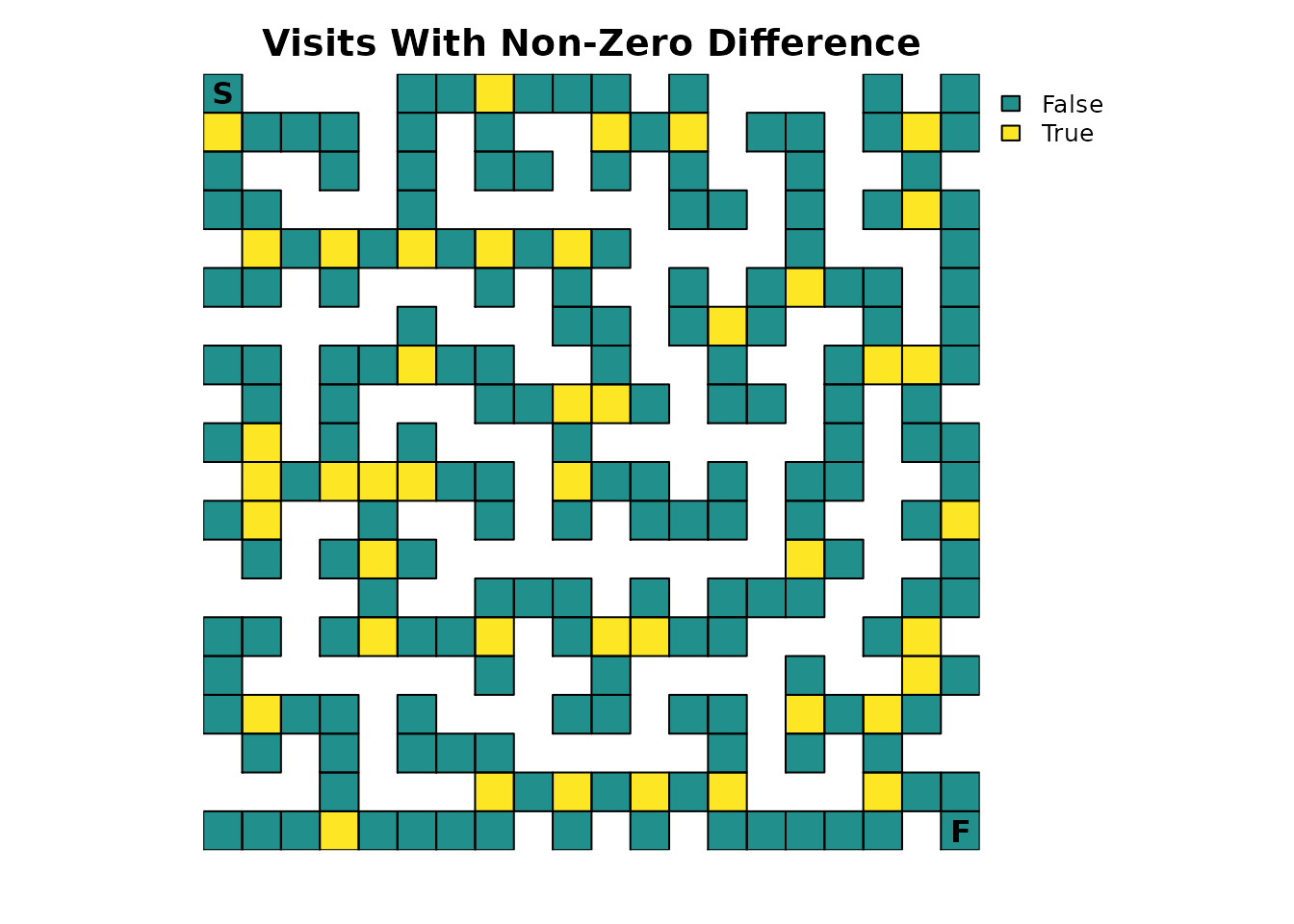It turns out that there is no change in the number of expected visits for non-intersections. It also turns out that our hunch for the intersections was only partially true; the change is constant, but it’s 1/9 instead of 0.1 or 10%.

The most interesting change from incorporating fidelity might be with the distribution() metric. Recall from Part 1 that there was an alternating pattern with the cells when changing the time steps. With fidelity, this effect still exists, but not to the same degree:

ints_dist <- distribution(ints_samc, origin = maze_origin, time = 20)
plot_maze(map(ints_samc, ints_dist), "Location at t=20", viridis(256))

ints_dist <- distribution(ints_samc, origin = maze_origin, time = 21)
plot_maze(map(ints_samc, ints_dist), "Location at t=21", viridis(256))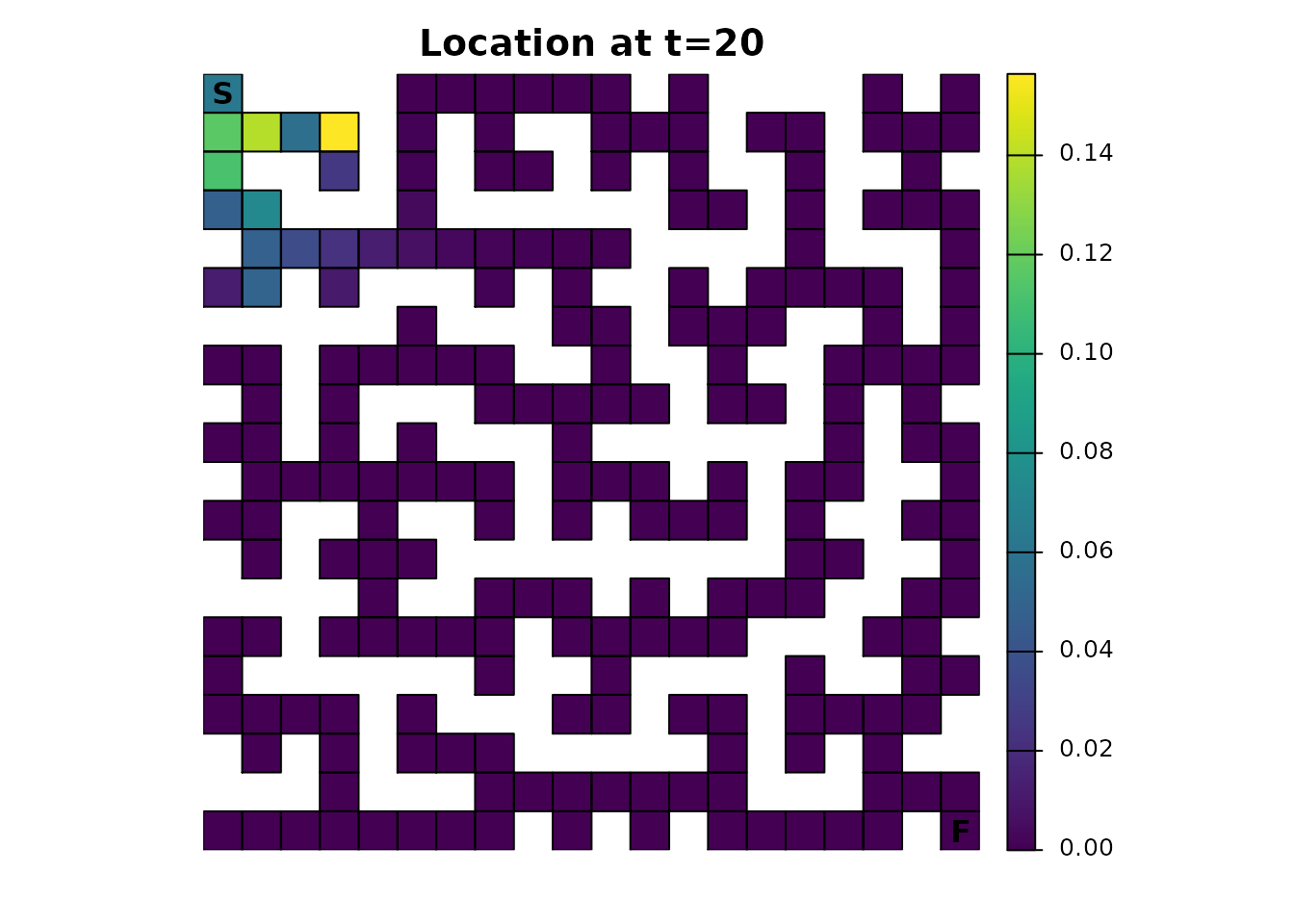Given a sufficient amount of time, the cumulative effect of having fidelity present will almost entirely eliminate this pattern. Even from time steps 200-201, the alternating pattern is visually nearly gone:

ints_dist <- distribution(ints_samc, origin = maze_origin, time = 200)
plot_maze(map(ints_samc, ints_dist), "Location at t=200", viridis(256))

ints_dist <- distribution(ints_samc, origin = maze_origin, time = 201)
plot_maze(map(ints_samc, ints_dist), "Location at t=201", viridis(256))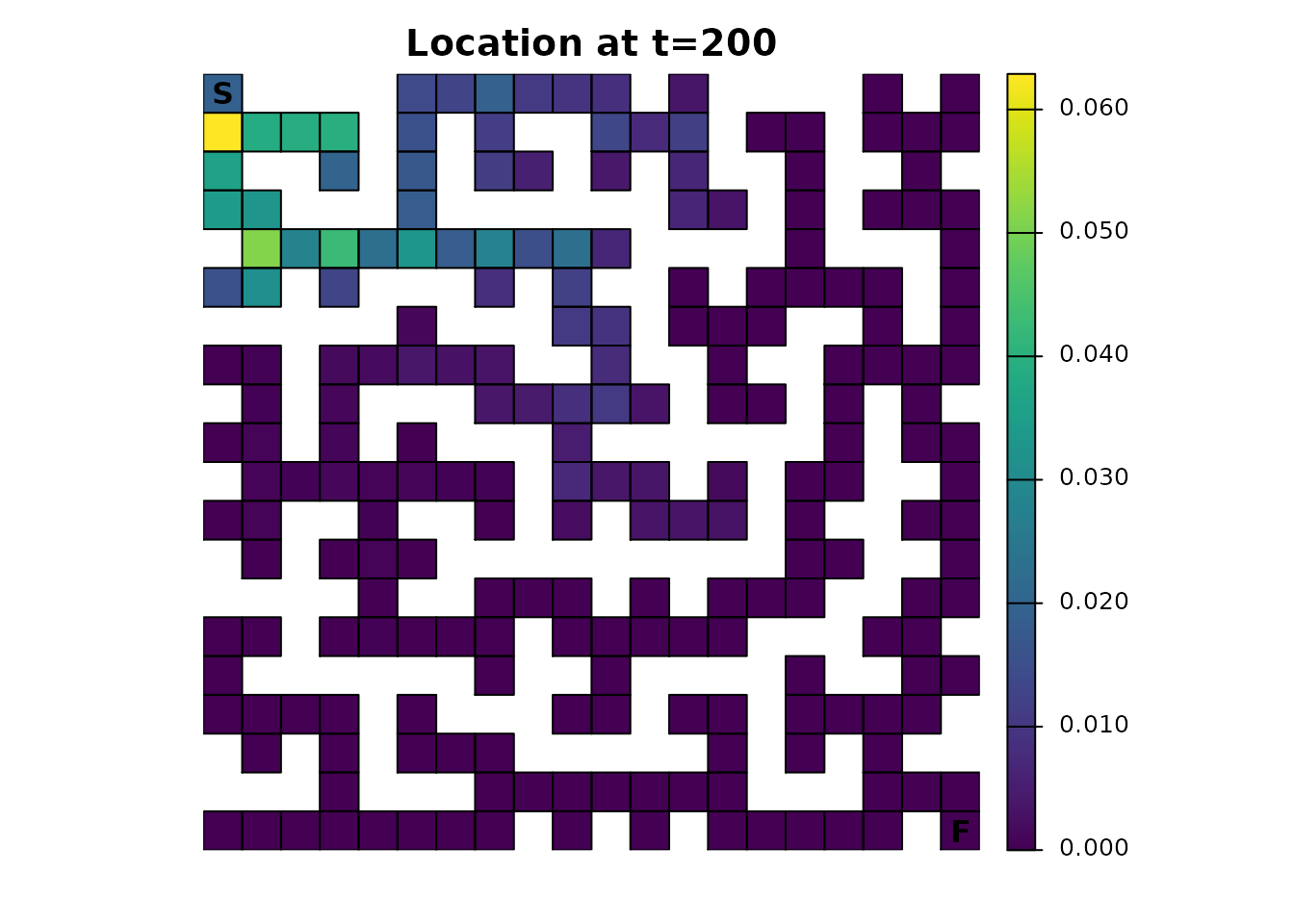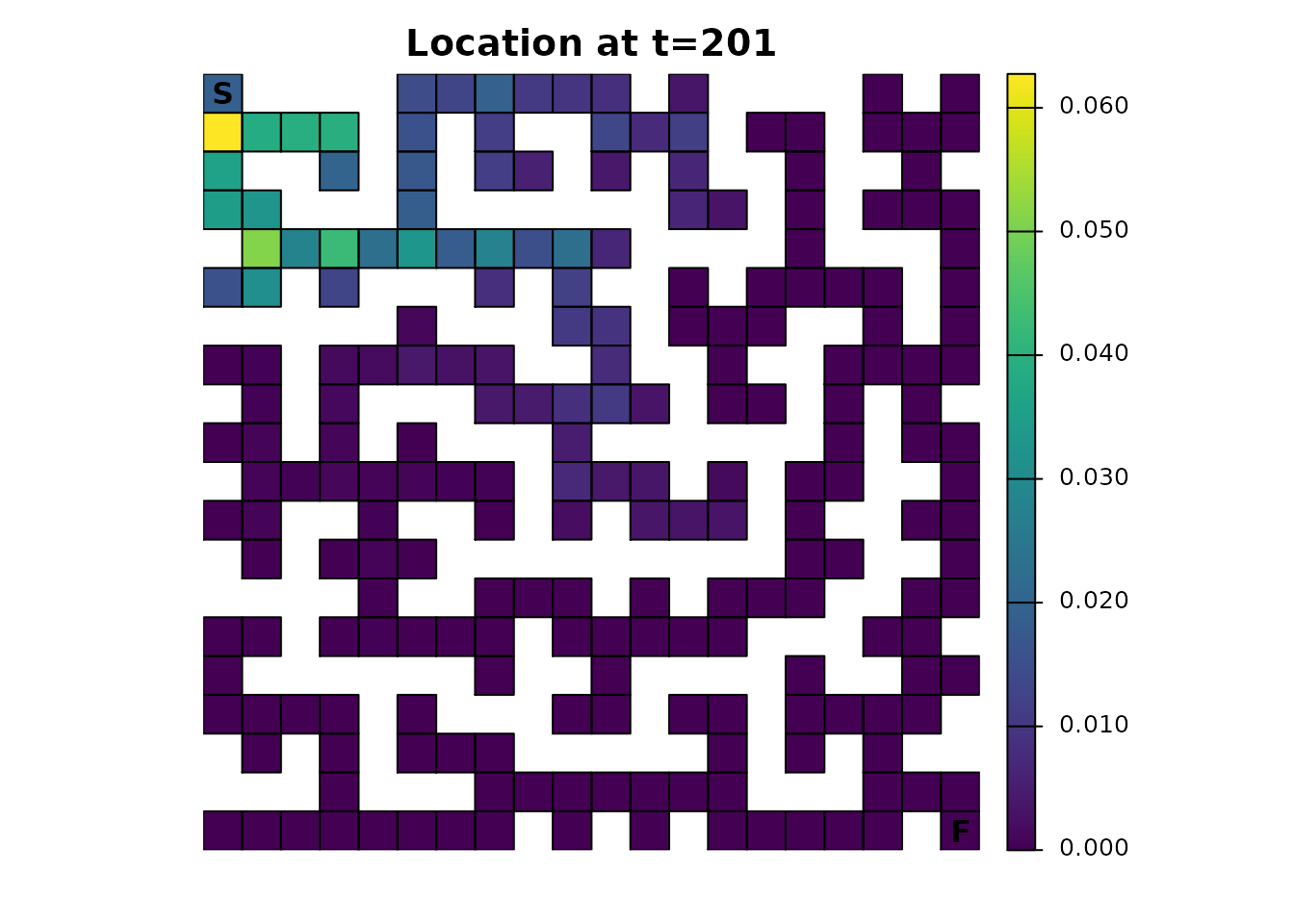For comparison, here’s the original samc object using the same time steps:

maze_dist <- distribution(maze_samc, origin = maze_origin, time = 200)
plot_maze(map(maze_samc, maze_dist), "Location at t=200", viridis(256))

maze_dist <- distribution(maze_samc, origin = maze_origin, time = 201)
plot_maze(map(maze_samc, maze_dist), "Location at t=201", viridis(256))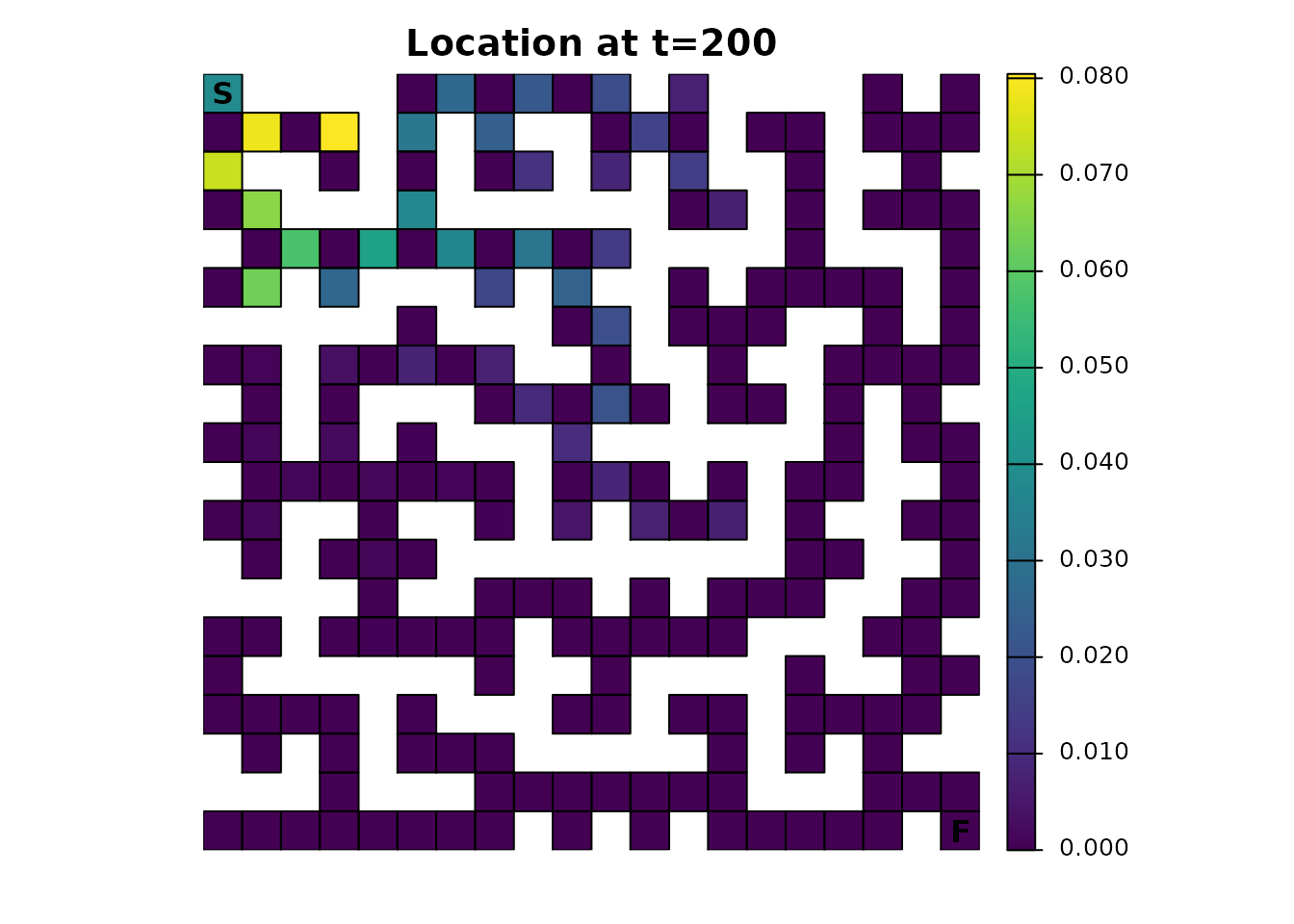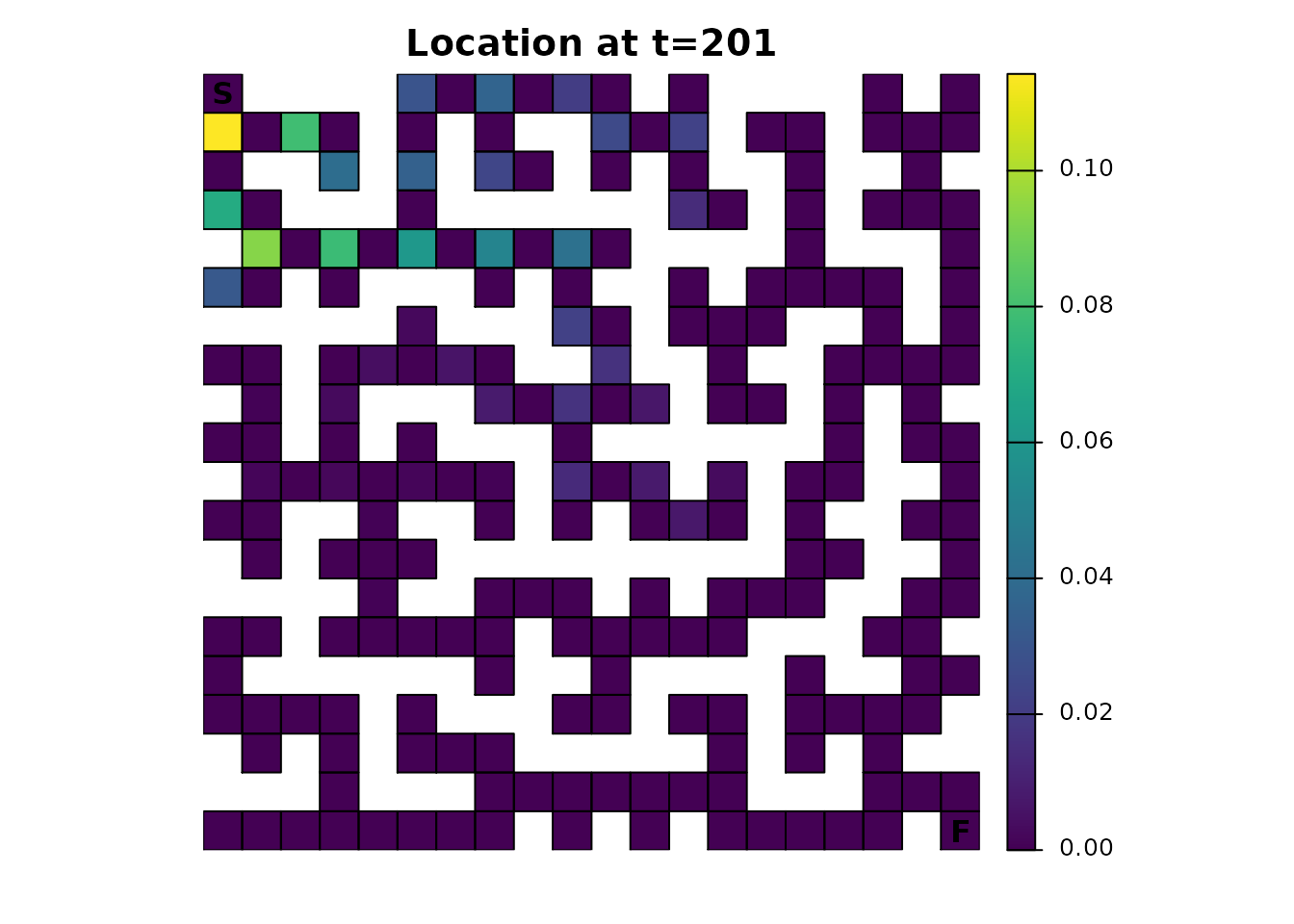Technically, the package doesn’t offer the ability to “look ahead” at future states to adjust the transition probabilities. In other words, if a route would eventually lead to a dead end, there’s nothing in the samc() function or the metric functions to account for that or model the possibility that an individual in the maze can see down a hallway. It can, however, be faked somewhat by adjusting the resistance map so that the dead ends have a much higher resistance. This will reduce the probability of an individual entering a dead end, almost as if they looked ahead. A sliding window function can be used to create this new map:

# Dead ends
ends_res <- focal(maze_res,
w = matrix(c(NA, 1, NA, 1, 1, 1, NA, 1, NA), nrow = 3, ncol = 3),
fun = function(x){sum(!is.na(x)) == 2})
ends_res[is.na(maze_res)] <- NA
ends_res <- ends_res * 9 + 1
ends_res[20, 20] <- 1

plot_maze(ends_res, "Dead Ends", vir_col)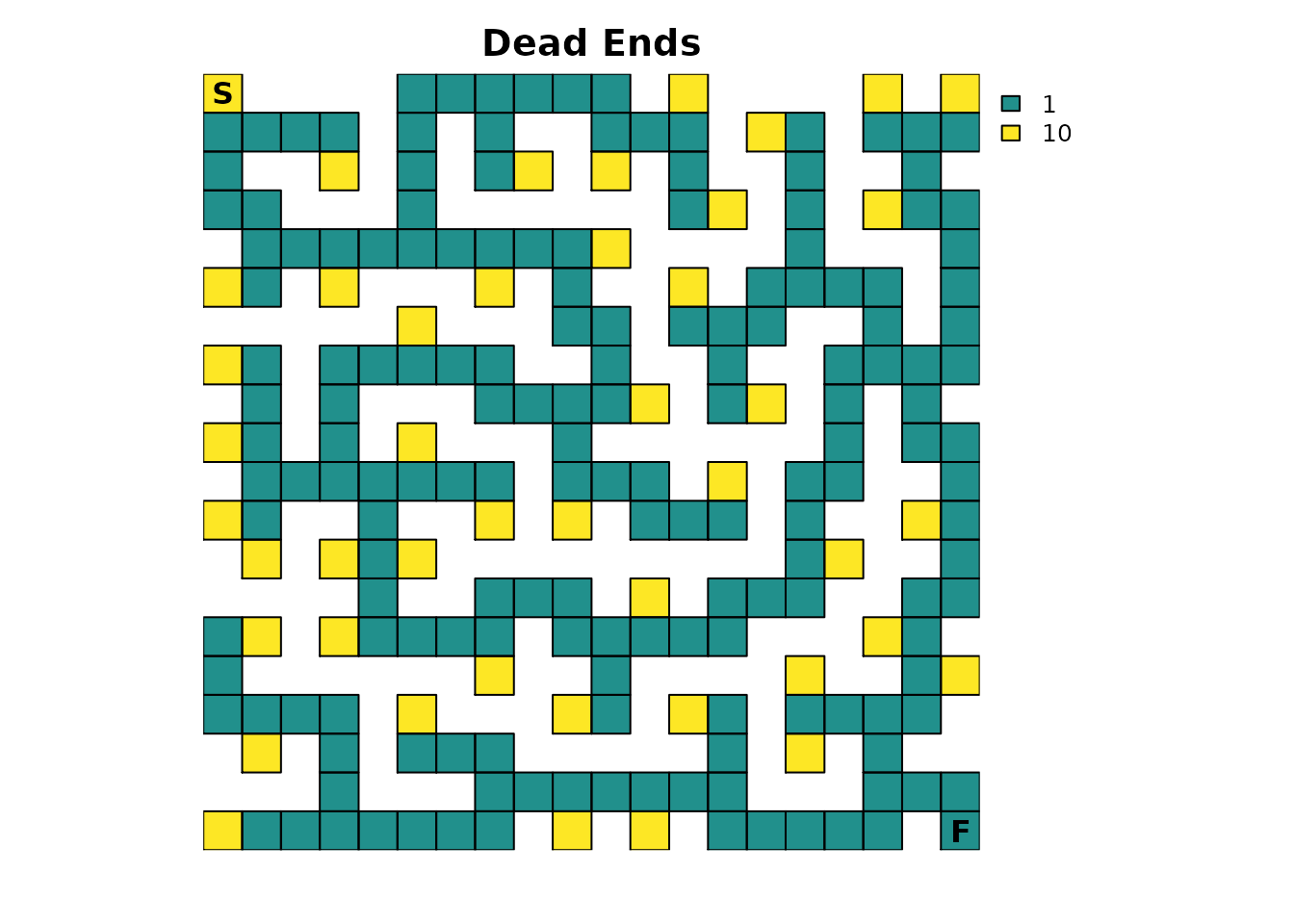The dead ends have been assigned a resistance value of 10, which is relatively high and means that dead ends will only rarely be entered. Since the resistance map has been modified, the samc object will need to be recreated. The fidelity data from the previous section will not be used, which will allow direct comparisons against the model created in Part 1.

ends_samc <- samc(ends_res, maze_finish, model = rw_model)

Hypothetically, since an individual can now “look ahead”, they should be able to get through the maze faster because they are spending less time in dead ends. This is easily verified:

# Original results from Part 1
survival(maze_samc)[maze_origin]
#>  13869
cond_passage(maze_samc, origin = maze_origin, dest = maze_dest)
#>  13868

survival(ends_samc)[maze_origin]
#>  11313
cond_passage(ends_samc, origin = maze_origin, dest = maze_dest)
#>  11312

Since the dead ends have a lower probability of being transitioned to, the dispersal() and visitation() metrics should reflect that:

ends_disp <- dispersal(ends_samc, origin = maze_origin)
#>
#> Performing setup. This can take several minutes... Complete.
#> Calculating matrix inverse diagonal...
#>
Computing: 100% (done)
#>
Complete
#> Diagonal has been cached. Continuing with metric calculation...
plot_maze(map(maze_samc, ends_disp), "Probability of Visit", viridis(256))

ends_visit <- visitation(ends_samc, origin = maze_origin)
plot_maze(map(maze_samc, ends_visit), "Visits Per Cell", viridis(256))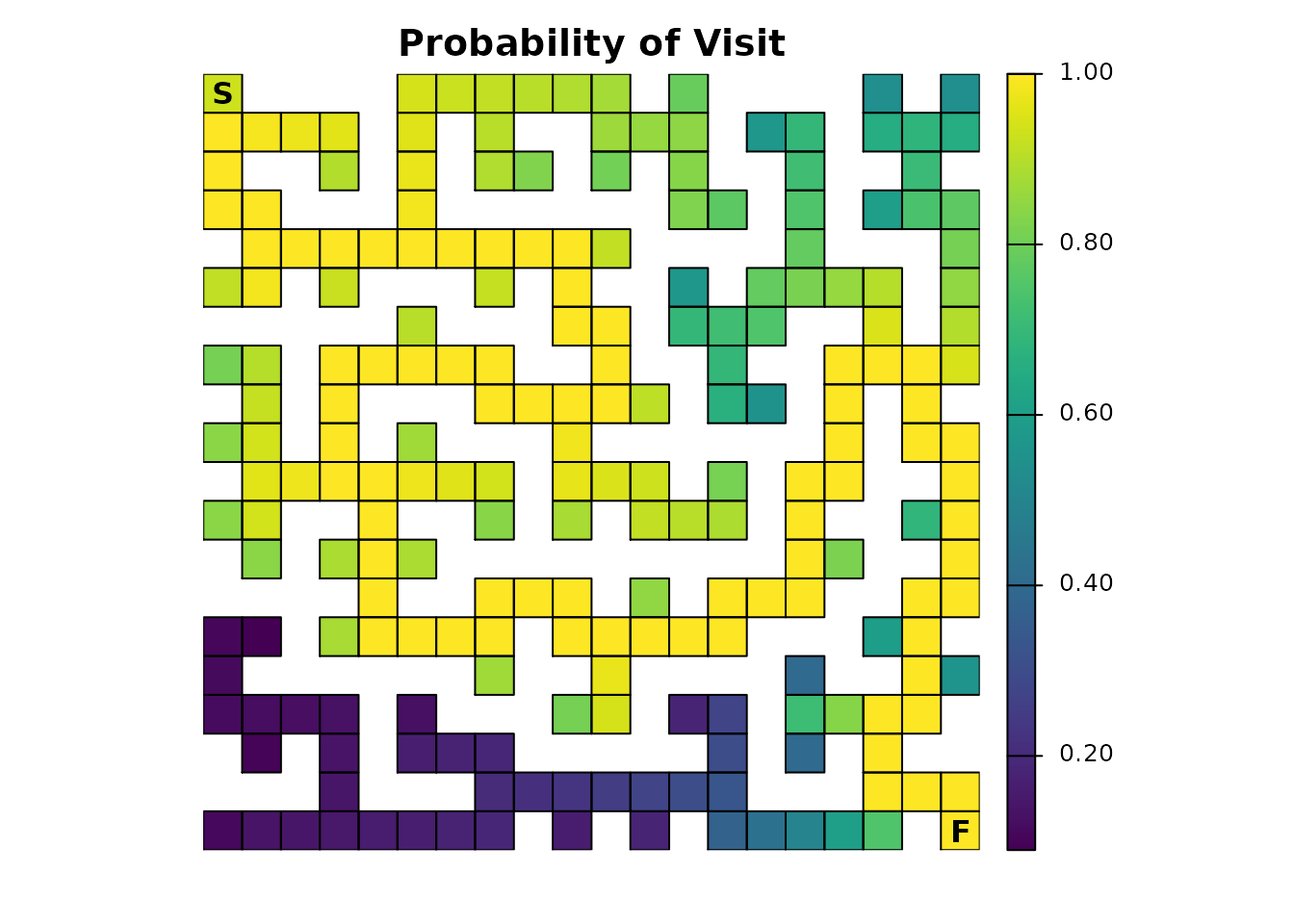The effect is more obvious with the expected number of visits from visitation(); the probability illustration is more subtle compared to the original results in Part 1. This could be explored similarly to how some of the differences in the fidelity section are illustrated, an exercise that will be left to interested readers.

## Traps

It’s fairly to trivial to add lethal traps to the maze by updating the absorption input to the samc() function. The key thing to keep in mind is that the samc() function expects the total absorption, so it will only be provided a single absorption input. However, the package can be used to tease apart the role that different sources of absorption will have in the model. There are two different approaches to setting this up:

1. Start with a single total absorption input. Then take that input and decompose it into multiple absorption components.
2. Start with multiple absorption components. Then take those inputs and combine them into a single total absorption input.

The choice depends on the data available and the goals of the project. For example, the first strategy is useful if we’ve somehow measured total absorption for a model and want to explore different hypotheses for how it breaks down into different types of absorption. The second is useful if we already have direct knowledge of different sources of absorption.

This example will take the second approach. One absorption component has already been created for the finish point. A second simple absorption component will be created that represents a few traps with a 0.2 or 20% absorption probability:

# Traps absorption layer
maze_traps <- maze_res * 0
maze_traps[17, 3] <- 0.2
maze_traps[1, 9] <- 0.2
maze_traps[6, 20] <- 0.2

plot_maze(maze_traps, "Traps", vir_col)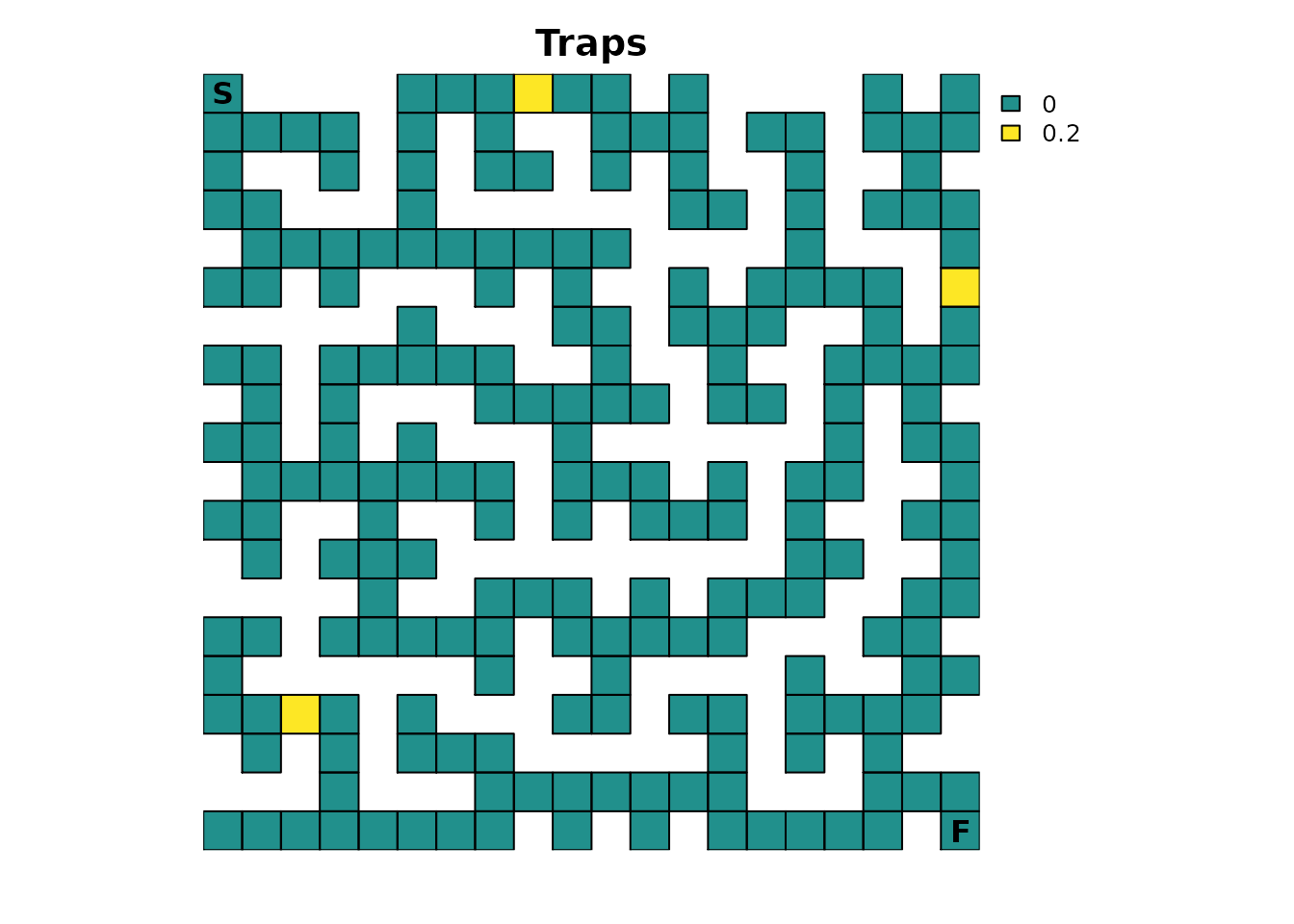Since the total absorption is the sum of these two components, the samc object will have to be recreated:

maze_abs_total <- maze_finish + maze_traps

traps_samc <- samc(maze_res, maze_abs_total, model = rw_model)

For easy comparison, everything else will be kept the same as the original example from Part 1. Continuing the previous strategy, let’s start with determining how long it is expected for an individual to finish the maze:

# Original results from Part 1
survival(maze_samc)[maze_origin]
#>  13869
cond_passage(maze_samc, origin = maze_origin, dest = maze_dest)
#>  13868

# Results with traps
survival(traps_samc)[maze_origin]
#>  1330.26
cond_passage(traps_samc, origin = maze_origin, dest = maze_dest)
#>  3060.207

The results are drastically different from what has been seen before. First, the clear relationship between survival() and cond_passage() no longer exists. This is because survival() has a different interpretation in this context and no longer determines how long it will take to finish; instead, it now calculates how long it will take an individual to either finish or be absorbed in one of the traps (i.e., die). This also drastically changes the plotting results of survival() (note the change in figure title from Part 1 to reflect the new interpretation):

traps_surv <- survival(traps_samc)

# Note the updated title from part 1
plot_maze(map(maze_samc, traps_surv), "Expected Time to Absorption", viridis(256))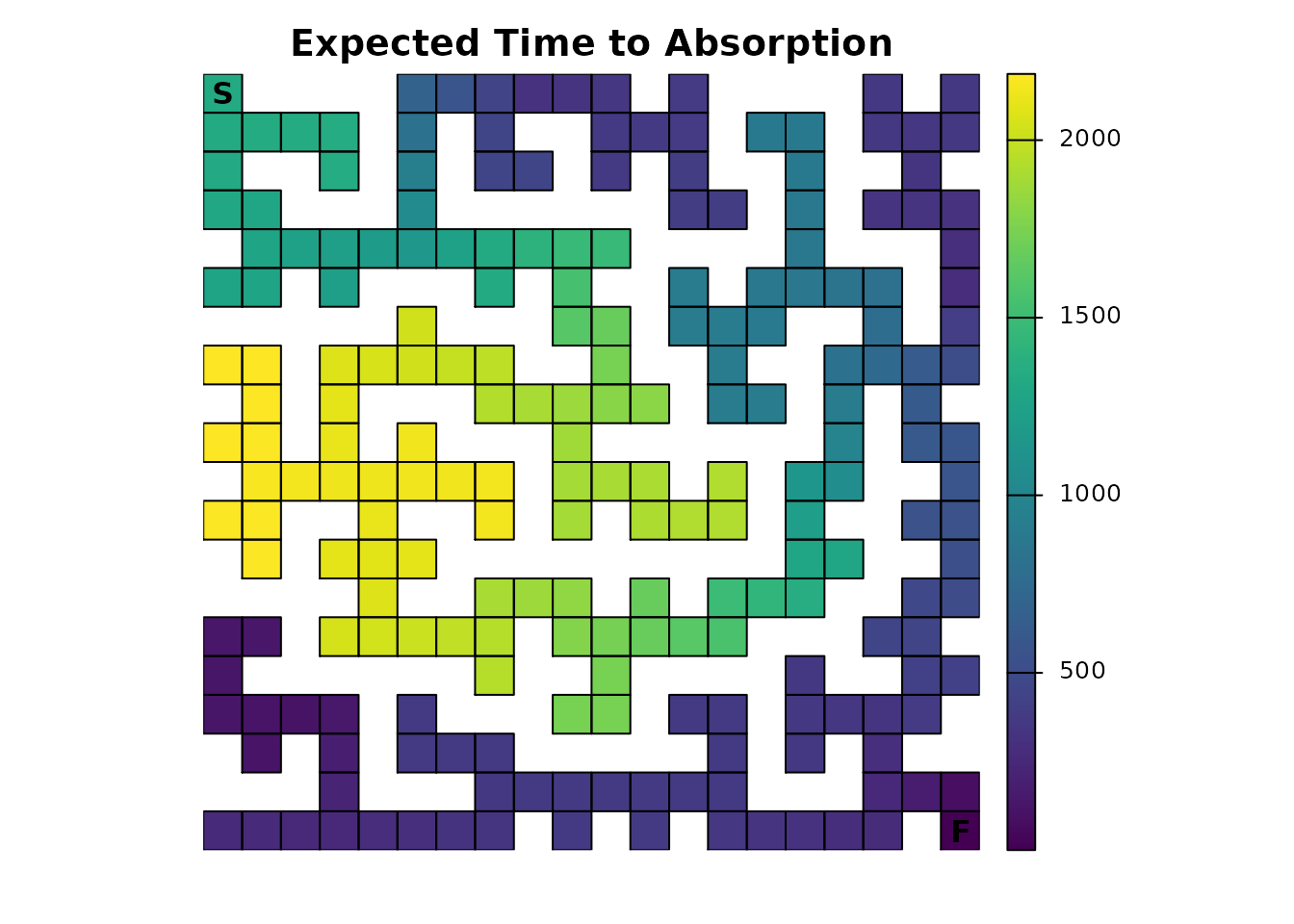The results are also drastically different from Part 1 when looking at visitation probability and the number of visits:

traps_disp <- dispersal(traps_samc, origin = maze_origin)
#>
#> Performing setup. This can take several minutes... Complete.
#> Calculating matrix inverse diagonal...
#>
Computing: 100% (done)
#>
Complete
#> Diagonal has been cached. Continuing with metric calculation...
plot_maze(map(traps_samc, traps_disp), "Probability of Visit", viridis(256))

traps_visit <- visitation(traps_samc, origin = maze_origin)
plot_maze(map(traps_samc, traps_visit), "Visits Per Cell", viridis(256))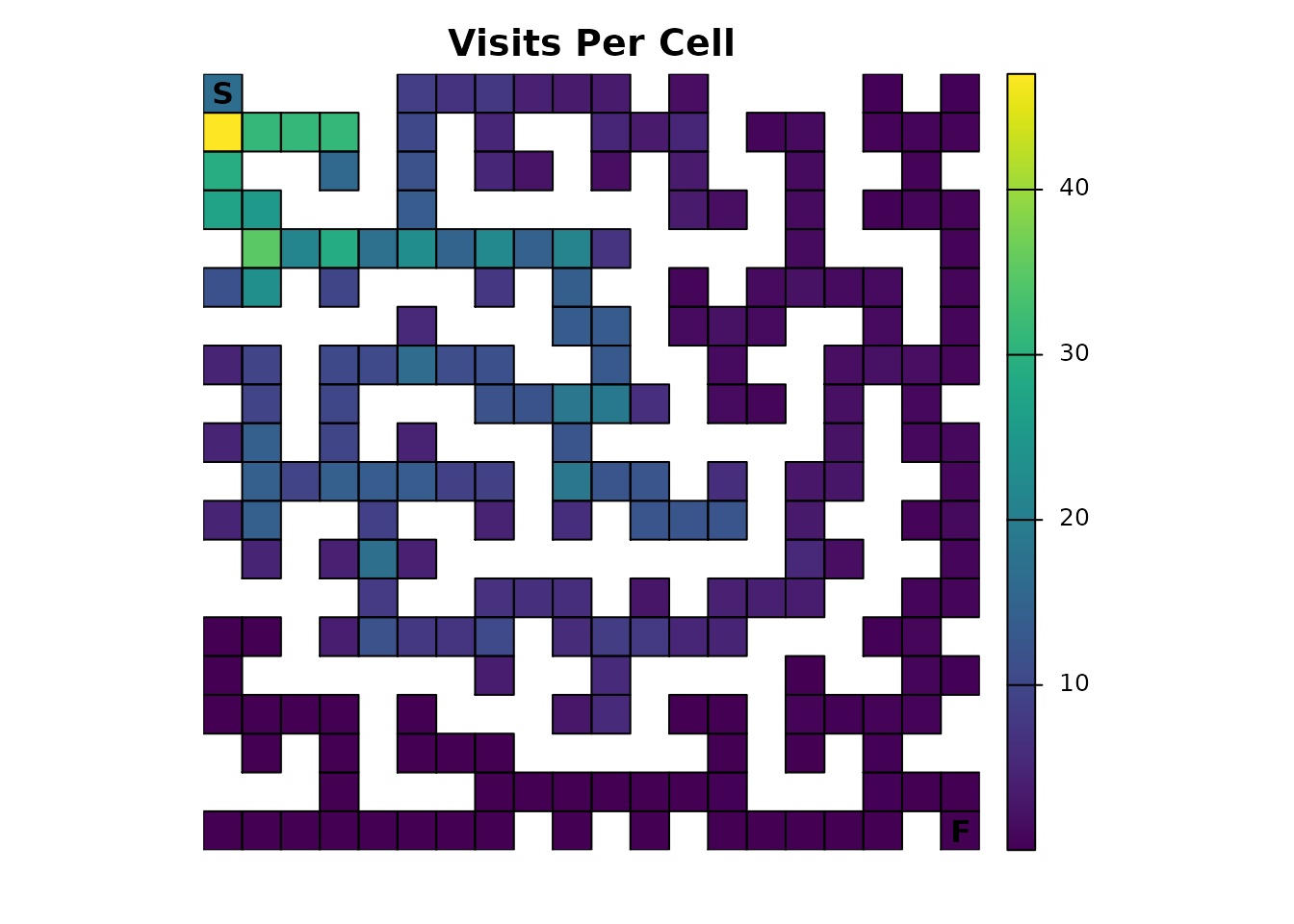Importantly, the technique in Part 1 of using visitation probabilities of 1.0 to identify the route through the maze will not work in this example; it only works in very specialized cases. The reason is simple: since an individual can now be absorbed in other locations, there is a non-zero probability that they never reach the finish, which in turn means the probability of visiting the finish is now less than 1.0. However, the same technique can be used to see something interesting:

# Ideally, we would just use as.numeric(traps_disp == 1), but we have floating point precision issues here, so we will approximate it
traps_disp_route <- as.numeric(abs(traps_disp - 1) < tolerance)

plot_maze(map(traps_samc, traps_disp_route), "dispersal() == 1", vir_col)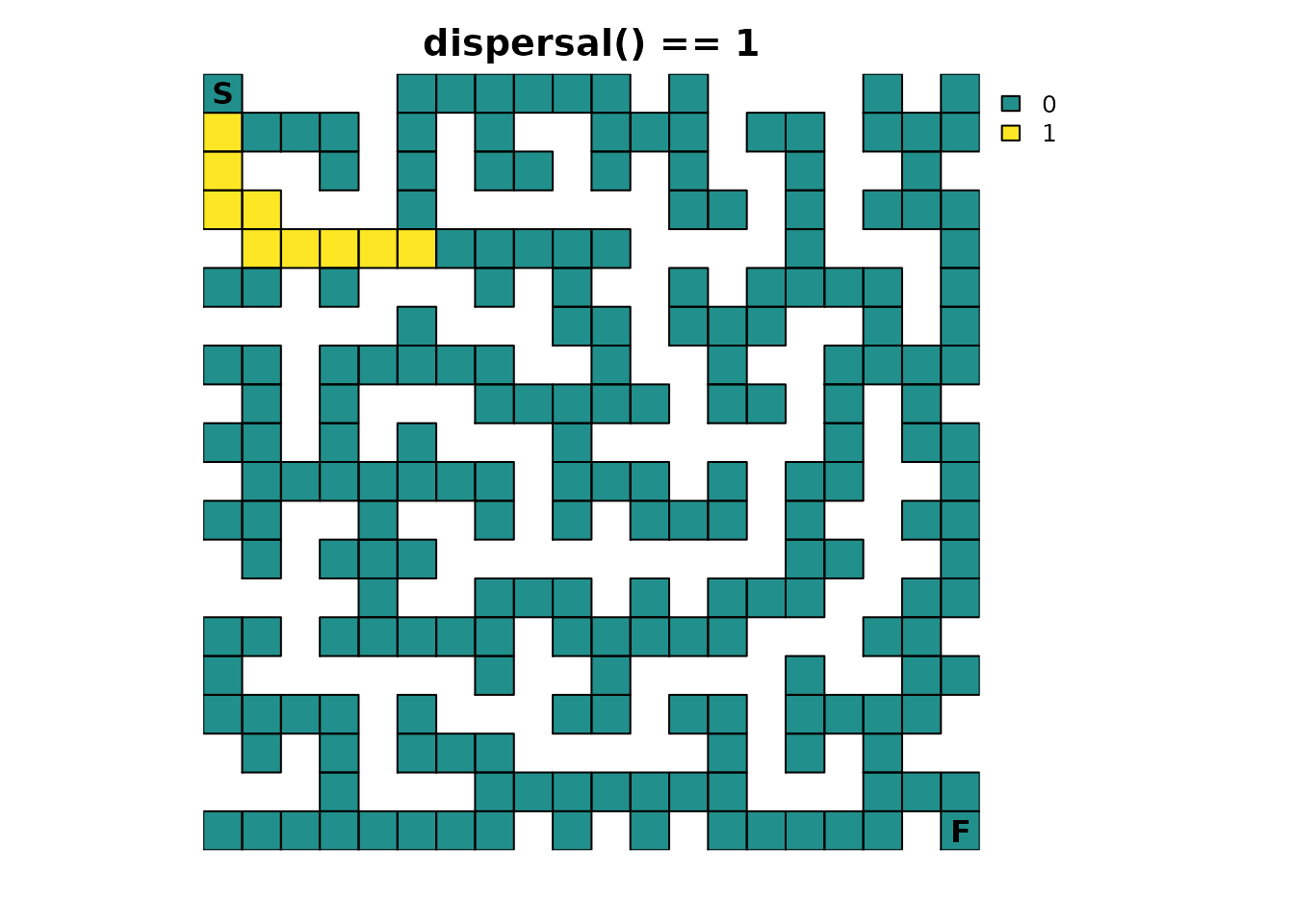It shows part of the solution observed before, but only up to the first maze intersection that leads to two or more possible sources of absorption.

The inclusion of multiple absorption states makes the metrics that were not useful in Part 1 more relevant. Starting with mortality(), it is possible to visualize where an individual is expected to be absorbed:

traps_mort <- mortality(traps_samc, origin = maze_origin)

plot_maze(map(traps_samc, traps_mort), "Absorption Probability", viridis(256))This result is quite possibly unexpected. Why does the finish point look like it’s 0? Looking at the numbers might provide insight:

traps_mort[traps_mort > 0]
#>  0.852084306 0.113761093 0.003940915 0.030213685

traps_mort[maze_dest]
#>  0.03021369

There’s only a 3.0% chance of an individual finishing the maze! This might seem low given the traps are only lethal 20% of the time, but it makes sense. Recall the Probability of visiting a cell and Visits per cell sections from Part 1; an individual spends most of their time in the early part of the maze. That means they have a lot more exposure to the first trap and, consequently, are more likely to be absorbed there with an 85.2% probability. For the trap farthest from the start, reaching it first requires passing by the finish, so consequently, it has only a 0.39% of being the source of absorption, a substantially lower probability than just finishing the maze.

It is possible to break down the total absorption so that the role of different sources of absorption can be investigated more easily. Now that the samc object has been created, it can be provided the original absorption layers that were used to calculate the total absorption:

# Naming the rasters will make things easier and less prone to user error later
names(maze_finish) <- "Finish"
names(maze_traps) <- "Traps"

traps_samc$abs_states <- c(maze_finish, maze_traps) By doing so, the mortality() metric now returns a list with information about not just the total absorption, but the individual components as well. This allows the role of different types of absorption to be individually accessed and visualized: traps_mort_dec <- mortality(traps_samc, origin = maze_origin) str(traps_mort_dec) #> List of 3 #>$ total : num [1:215] 0 0 0 0 0.852 ...
#>  $Finish: num [1:215] 0 0 0 0 0 0 0 0 0 0 ... #>$ Traps : num [1:215] 0 0 0 0 0.852 ...

plot_maze(map(traps_samc, traps_mort_dec$Finish), "Absorption Probability (Finish)", viridis(256)) plot_maze(map(traps_samc, traps_mort_dec$Traps), "Absorption Probability (Traps)", viridis(256))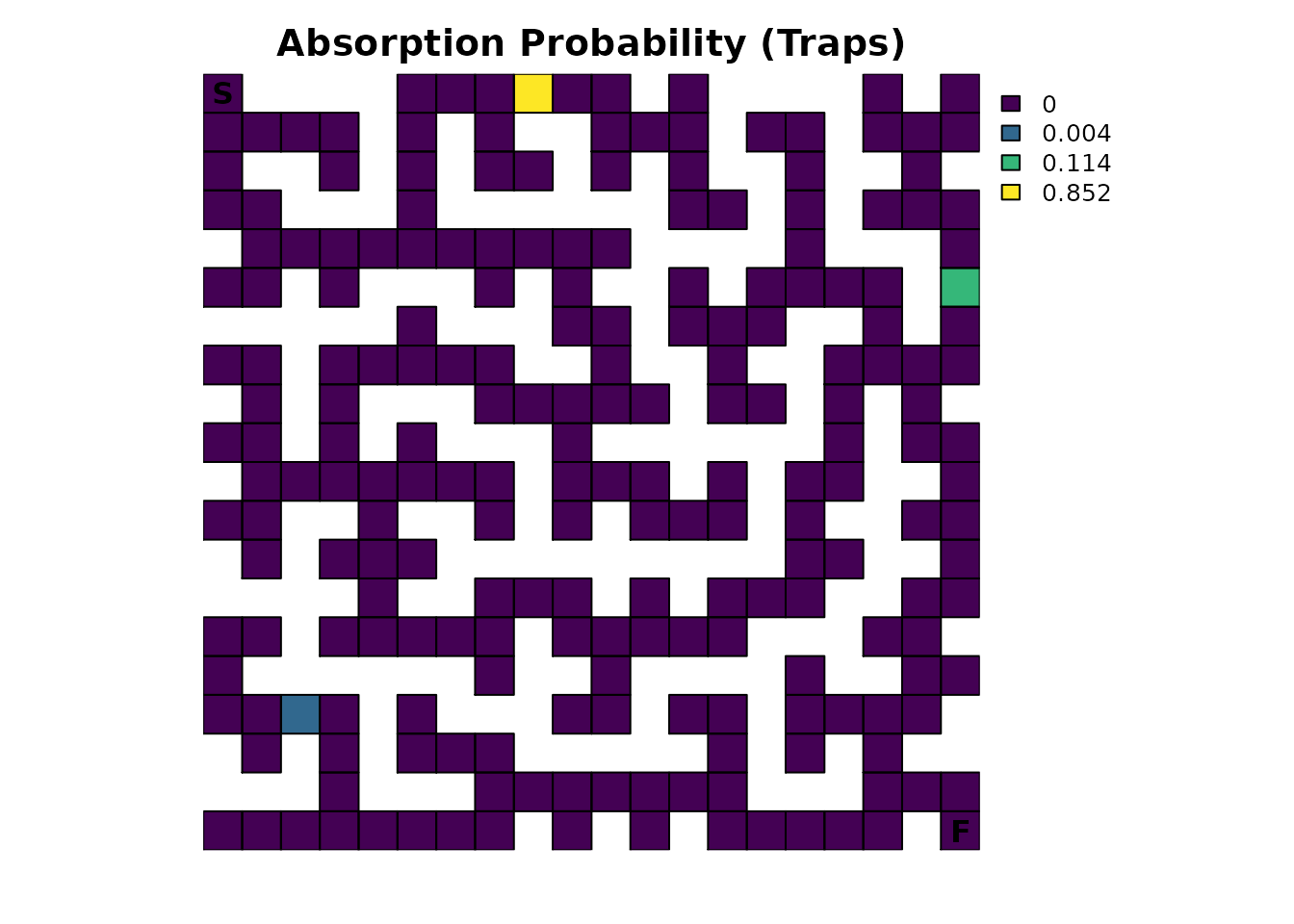With multiple sources of absorption now specified in the samc object, the absorption() metric becomes relevant:

absorption(traps_samc, origin = maze_origin)
#>     Finish      Traps
#> 0.03021369 0.96978631

The output from this is quite simple: it is the probability that an individual will experience a particular type of absorption. As seen before, there is a 3.0% chance of finishing the maze. But absorption() also shows that there is a 97.0% total probability that absorption will occur in one of the three traps. This is different from the mortality() metric, which calculates the absorption probabilities at each cell. There is clearly a relationship between the two metrics, and the advantage of this example is that it’s easy to see it; it is more difficult to see how the two metrics are related in more complex situations.

## Part 3

The next part of this example series will take the maze with only a single solution and modify it with a “secret” shortcut. Wrapping up this example, Part 3 will then take all of the changes introduced in the series and combine them into a single final example.